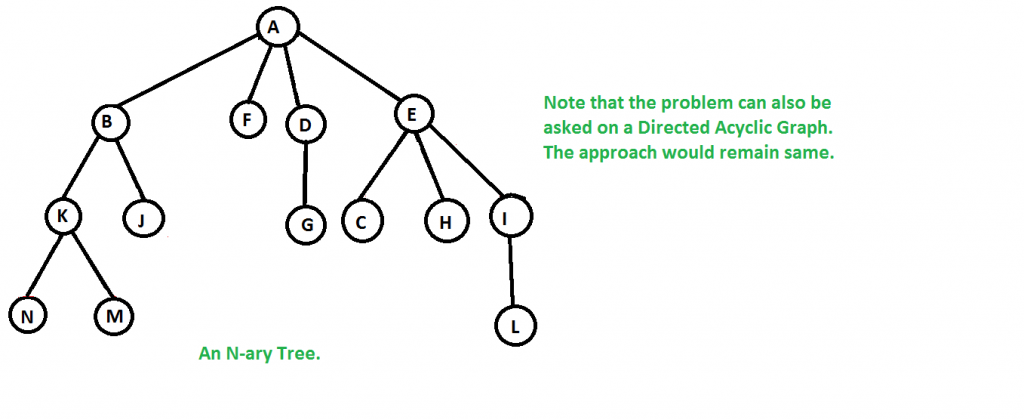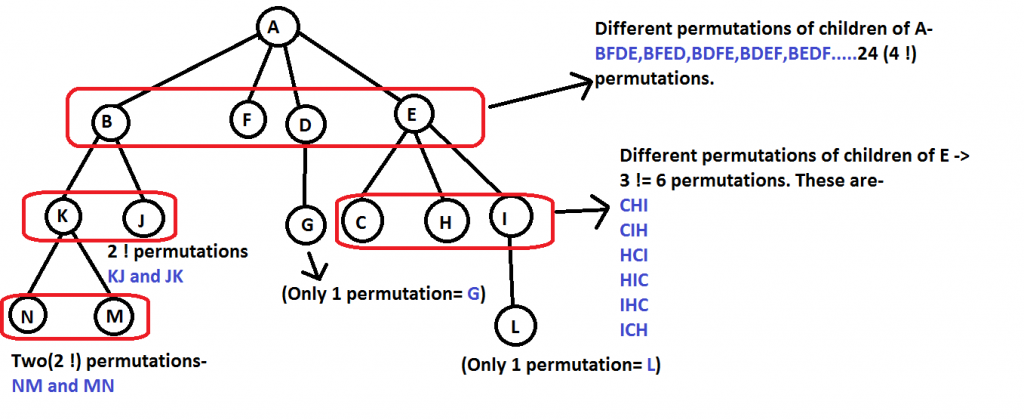GeeksforGeeks App
Open AppBrowser
Continue

# Number of ways to traverse an N-ary tree

Given an n-ary tree, count the number of ways to traverse an n-ary (or a Directed Acyclic Graph) tree starting from the root vertex

Example:

Suppose we have a given N-ary tree as shown below:Now we have to find the number of ways of traversing the whole tree starting from the root vertex. There can be many such ways. Some of them are listed below.

• N->M->K->J->B->F->D->E->C->H->I->L->A (kind-of depth-first traversal)
• A->B->F->D->E->K->J->G->C->H->I->N->M->L (level order traversal)

Note: The count of all ways to traverse is the product of factorials of the number of children of each node. Refer to the below figure for a clear understanding:Here,
‘A’ has four children, so 4! permutations possible
‘B’ has two children, so 2! permutations possible
‘F’ has no children, so 0! permutations possible
…..
And so on
Hence all such ways are- 4 ! * 2 ! * 0 ! * 1 ! * 3 ! * 2 ! * 0 ! * 0 ! * 0 ! * 0 ! * 1 ! * 0 ! * 0 ! = 576 way
That’s a huge number of ways and among them, only few prove to be useful, like- inorder, level-order, preorder, and postorder (arranged according to the popularity of these traversals)

Follow the below steps to solve the problem:

• Initialize ways equal to one and create a queue of type Node and push root into it
• While the queue is not empty
• Initialize integer p equal to the front Node of the queue
• Set ways equal to ways * x!, where x is the count of p’s children
• Enqueue all the children of the Node p
• Return ways

Below is the implementation of the above approach:

## C++

 `// C++ program to find the number of ways to traverse a``// n-ary tree starting from the root node``#include ``using` `namespace` `std;` `// Structure of a node of an n-ary tree``struct` `Node {``    ``char` `key;``    ``vector child;``};` `// Utility function to create a new tree node``Node* newNode(``int` `key)``{``    ``Node* temp = ``new` `Node;``    ``temp->key = key;``    ``return` `temp;``}` `// Utility Function to find factorial of given number``int` `factorial(``int` `n)``{``    ``if` `(n == 0)``        ``return` `1;``    ``return` `n * factorial(n - 1);``}` `// Function to calculate the number of ways of traversing``// the n-ary starting from root.``// This function is just a modified breadth-first search.``// We can use a depth-first search too.``int` `calculateWays(Node* root)``{``    ``int` `ways = 1; ``// Initialize result` `    ``// If the tree is empty there is no way of traversing``    ``// the tree.``    ``if` `(root == NULL)``        ``return` `0;` `    ``// Create a queue and enqueue root to it.``    ``queue q;``    ``q.push(root);` `    ``// Level order traversal.``    ``while` `(!q.empty()) {``        ``// Dequeue an item from queue and print it``        ``Node* p = q.front();``        ``q.pop();` `        ``// The number of ways is the product of``        ``// factorials of number of children of each node.``        ``ways = ways * (factorial(p->child.size()));` `        ``// Enqueue all childrent of the dequeued item``        ``for` `(``int` `i = 0; i < p->child.size(); i++)``            ``q.push(p->child[i]);``    ``}` `    ``return` `(ways);``}` `// Driver code``int` `main()``{``    ``/*   Let us create below tree``     ``*           A``     ``*         / /  \  \``     ``*       B  F   D  E``     ``*      / \     |  /|\``     ``*     K  J    G  C H I``     ``*      /\            \``     ``*    N   M            L``     ``*/` `    ``Node* root = newNode(``'A'``);``    ``(root->child).push_back(newNode(``'B'``));``    ``(root->child).push_back(newNode(``'F'``));``    ``(root->child).push_back(newNode(``'D'``));``    ``(root->child).push_back(newNode(``'E'``));``    ``(root->child->child).push_back(newNode(``'K'``));``    ``(root->child->child).push_back(newNode(``'J'``));``    ``(root->child->child).push_back(newNode(``'G'``));``    ``(root->child->child).push_back(newNode(``'C'``));``    ``(root->child->child).push_back(newNode(``'H'``));``    ``(root->child->child).push_back(newNode(``'I'``));``    ``(root->child->child->child)``        ``.push_back(newNode(``'N'``));``    ``(root->child->child->child)``        ``.push_back(newNode(``'M'``));``    ``(root->child->child->child)``        ``.push_back(newNode(``'L'``));` `    ``cout << calculateWays(root);``    ``;` `    ``return` `0;``}`

## Java

 `//Java code for the above approach``import` `java.util.ArrayList;``import` `java.util.LinkedList;``import` `java.util.Queue;``public` `class` `GFG {``    ``// Structure of a node of an n-ary tree``    ``static` `class` `Node {``        ``char` `key;``        ``ArrayList child;``        ``public` `Node(``char` `key)``        ``{``            ``this``.key = key;``            ``child = ``new` `ArrayList<>();``        ``}``    ``}` `    ``// Utility function to create a new tree node``    ``static` `Node newNode(``char` `key) { ``return` `new` `Node(key); }` `    ``// Utility Function to find factorial of given number``    ``static` `int` `factorial(``int` `n)``    ``{``        ``if` `(n == ``0``) {``            ``return` `1``;``        ``}``        ``return` `n * factorial(n - ``1``);``    ``}` `    ``// Function to calculate the number of ways of``    ``// traversing the n-ary starting from root. This``    ``// function is just a modified breadth-first search. We``    ``// can use a depth-first search too.``    ``static` `int` `calculateWays(Node root)``    ``{``        ``int` `ways = ``1``; ``// Initialize result` `        ``// If the tree is empty there is no way of``        ``// traversing the tree.``        ``if` `(root == ``null``) {``            ``return` `0``;``        ``}` `        ``// Create a queue and enqueue root to it.``        ``Queue q = ``new` `LinkedList<>();``        ``q.add(root);` `        ``// Level order traversal.``        ``while` `(!q.isEmpty()) {``            ``// Dequeue an item from queue and print it``            ``Node p = q.poll();` `            ``// The number of ways is the product of``            ``// factorials of number of children of each``            ``// node.``            ``ways = ways * (factorial(p.child.size()));` `            ``// Enqueue all children of the dequeued item``            ``for` `(``int` `i = ``0``; i < p.child.size(); i++) {``                ``q.add(p.child.get(i));``            ``}``        ``}` `        ``return` `(ways);``    ``}` `    ``// Driver code``    ``public` `static` `void` `main(String[] args)``    ``{``        ``/*   Let us create below tree``         ``*           A``         ``*         / /  \  \``         ``*       B  F   D  E``         ``*      / \     |  /|\``         ``*     K  J    G  C H I``         ``*      /\            \``         ``*    N   M            L``         ``*/` `        ``Node root = newNode(``'A'``);``        ``root.child.add(newNode(``'B'``));``        ``root.child.add(newNode(``'F'``));``        ``root.child.add(newNode(``'D'``));``        ``root.child.add(newNode(``'E'``));``        ``root.child.get(``0``).child.add(newNode(``'K'``));``        ``root.child.get(``0``).child.add(newNode(``'J'``));``        ``root.child.get(``2``).child.add(newNode(``'G'``));``        ``root.child.get(``3``).child.add(newNode(``'C'``));``        ``root.child.get(``3``).child.add(newNode(``'H'``));``        ``root.child.get(``3``).child.add(newNode(``'I'``));``        ``root.child.get(``0``).child.get(``0``).child.add(``            ``newNode(``'N'``));``        ``root.child.get(``0``).child.get(``0``).child.add(``            ``newNode(``'M'``));``        ``root.child.get(``3``).child.get(``2``).child.add(``            ``newNode(``'L'``));` `        ``System.out.println(calculateWays(root));``    ``}``}`

## Python3

 `# Python3 program to find the``# number of ways to traverse a``# n-ary tree starting from the root node`  `class` `Node:``    ``def` `__init__(``self``, key):``        ``self``.child ``=` `[]``        ``self``.key ``=` `key` `# Utility function to create a new tree node`  `def` `newNode(key):``    ``temp ``=` `Node(key)``    ``return` `temp` `# Utility Function to find factorial of given number`  `def` `factorial(n):` `    ``if` `(n ``=``=` `0``):``        ``return` `1` `    ``return` `n``*``factorial(n``-``1``)` `# Function to calculate the number of ways of traversing``# the n-ary starting from root.``# self function is just a modified breadth-first search.``# We can use a depth-first search too.`  `def` `calculateWays(root):` `    ``ways ``=` `1`  `# Initialize result` `    ``# If the tree is empty there is no way of traversing``    ``# the tree.``    ``if` `(root ``=``=` `None``):``        ``return` `0` `    ``# Create a queue and enqueue root to it.``    ``q ``=` `[]``    ``q.append(root)` `    ``# Level order traversal.``    ``while` `(``len``(q) > ``0``):``        ``# Dequeue an item from queue and print it``        ``p ``=` `q[``0``]``        ``q ``=` `q[``1``:]` `        ``# The number of ways is the product of``        ``# factorials of number of children of each node.``        ``ways ``=` `ways``*``(factorial(``len``(p.child)))` `        ``# Enqueue all childrent of the dequeued item``        ``for` `i ``in` `range``(``len``(p.child)):``            ``q.append(p.child[i])` `    ``return``(ways)` `#  Let us create below tree``# *         A``# *         / / \ \``# *     B F D E``# *     / \     | /|\``# *     K J G C H I``# *     /\         \``# * N M         L`  `root ``=` `newNode(``'A'``)``(root.child).append(newNode(``'B'``))``(root.child).append(newNode(``'F'``))``(root.child).append(newNode(``'D'``))``(root.child).append(newNode(``'E'``))``(root.child[``0``].child).append(newNode(``'K'``))``(root.child[``0``].child).append(newNode(``'J'``))``(root.child[``2``].child).append(newNode(``'G'``))``(root.child[``3``].child).append(newNode(``'C'``))``(root.child[``3``].child).append(newNode(``'H'``))``(root.child[``3``].child).append(newNode(``'I'``))``(root.child[``0``].child[``0``].child).append(newNode(``'N'``))``(root.child[``0``].child[``0``].child).append(newNode(``'M'``))``(root.child[``3``].child[``2``].child).append(newNode(``'L'``))` `print``(calculateWays(root))`

## C#

 `//C# code for the above approach``using` `System;``using` `System.Collections.Generic;` `class` `Node``{``    ``public` `char` `Key { ``get``; ``set``; }``    ``public` `List Children { ``get``; ``set``; }` `    ``public` `Node(``char` `key)``    ``{``        ``Key = key;``        ``Children = ``new` `List();``    ``}``}` `class` `Program``{``     ``// Utility Function to find factorial of given number``    ``static` `int` `Factorial(``int` `n)``    ``{``        ``if` `(n == 0)``        ``{``            ``return` `1;``        ``}` `        ``return` `n * Factorial(n - 1);``    ``}``    ``// Function to calculate the number of ways of``    ``// traversing the n-ary starting from root. This``    ``// function is just a modified breadth-first search. We``    ``// can use a depth-first search too.``    ``static` `int` `CalculateWays(Node root)``    ``{``        ``int` `ways = 1;  ``// Initialize result` `        ``// If the tree is empty there is no way of``        ``// traversing the tree.``        ``if` `(root == ``null``)``        ``{``            ``return` `0;``        ``}` `        ``Queue queue = ``new` `Queue();``        ``queue.Enqueue(root);` `        ``while` `(queue.Count != 0)``        ``{``            ``Node current = queue.Dequeue();``            ``ways *= Factorial(current.Children.Count);` `            ``foreach` `(Node child ``in` `current.Children)``            ``{``                ``queue.Enqueue(child);``            ``}``        ``}` `        ``return` `ways;``    ``}``    ``// Driver code``    ``static` `void` `Main(``string``[] args)``    ``{``        ``Node root = ``new` `Node(``'A'``);``        ``root.Children.Add(``new` `Node(``'B'``));``        ``root.Children.Add(``new` `Node(``'F'``));``        ``root.Children.Add(``new` `Node(``'D'``));``        ``root.Children.Add(``new` `Node(``'E'``));``        ``root.Children.Children.Add(``new` `Node(``'K'``));``        ``root.Children.Children.Add(``new` `Node(``'J'``));``        ``root.Children.Children.Add(``new` `Node(``'G'``));``        ``root.Children.Children.Add(``new` `Node(``'C'``));``        ``root.Children.Children.Add(``new` `Node(``'H'``));``        ``root.Children.Children.Add(``new` `Node(``'I'``));``        ``root.Children.Children.Children.Add(``new` `Node(``'N'``));``        ``root.Children.Children.Children.Add(``new` `Node(``'M'``));``        ``root.Children.Children.Children.Add(``new` `Node(``'L'``));` `        ``Console.WriteLine(CalculateWays(root));``    ``}``}`

## Javascript

 ``

Output

`576`

Time Complexity: O(N2). We can optimize the solution to work in O(N) time by per-computing factorials of all numbers from 1 to N
Auxiliary Space: O(N)

Common Pitfalls:
Since products of factorials can tend to grow very huge, so it may overflow. It is preferable to use data types like- unsigned long long int in C/C++, as the number of ways can never be a negative number. In Java and Python, there are Big Integers to take care of overflows

This article is contributed by Rachit Belwariar. If you like GeeksforGeeks and would like to contribute, you can also write an article and mail your article to review-team@geeksforgeeks.org. See your article appearing on the GeeksforGeeks main page and help other Geeks.

My Personal Notes arrow_drop_up